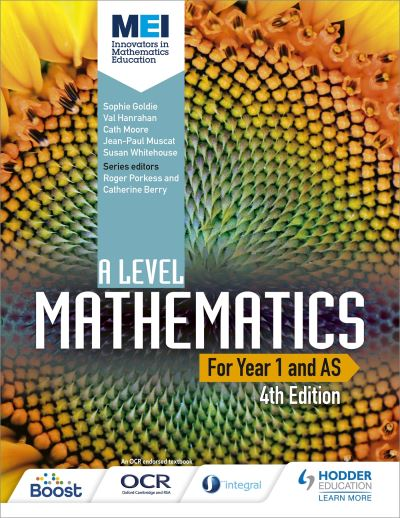# MEI A Level Mathematics for Year 1 and AS: student book : fourth edition

Series: MEI

Format: Paperback

ISBN: 9781471852978

Publication Date: 24 Feb 2017

Subject: Mathematics

Suitable for: Secondary (Year 12, Year 13)

Encourage every student to develop a deeper understanding of mathematical concepts and their applications with textbooks that draw on the well-known MEI (Mathematics in Education and Industry) series, updated and tailored to the 2017 OCR (MEI) specification and developed by subject specialists and MEI.

### £24.89

RRP £29.99 Save 17%

Quantity

• Our review
• Details
Notes
to view our full review.

Summary

Encourage every student to develop a deeper understanding of mathematical concepts and their applications with textbooks that draw on the well-known MEI (Mathematics in Education and Industry) series, updated and tailored to the 2017 OCR (MEI) specification and developed by subject specialists and MEI.

Endorsed by OCR.

-Develop problem-solving, proof and modelling skills with plenty of
questions and well-structured exercises that build skills and
mathematical techniques.
-Build connections between topics, using real-world contexts to help
develop mathematical modelling skills, thus providing a fuller and more
coherent understanding of mathematical concepts.
-Prepare students for assessment with practice questions written by
subject specialists.
-Ensure coverage of the new statistics requirements with five dedicated
statistics chapters and questions around the use of large data sets.
-Supports the use of technology with a variety of questions based around
the use of spreadsheets, graphing software and graphing calculators.
-Provide clear paths of progression that combine pure and applied maths
into a coherent whole.

Contents

Getting the most from this book
Prior knowledge

1 Problem solving
1.1 Solving problems
1.2 Writing mathematics
1.3 Proof
Problem solving: Mountain modelling
2 Surds and indices
2.1 Using and manipulating surds
2.2 Working with indices
3.2 The completed square form
4 Equations and inequalities
4.1 Simultaneous equations
4.2 Inequalities
5 Coordinate geometry
5.1 Working with coordinates
5.2 The equation of a straight line
5.3 The intersection of two lines
5.4 The circle
5.5 The intersection of a line and a curve
Problem solving: Integer point circles
Practice questions: Pure Mathematics 1
6 Trigonometry
6.1 Trigonometric functions
6.2 Trigonometric functions for angles of any size
6.3 Solving equations using graphs of trigonometric functions
6.4 Triangles without right angles
6.5 The area of a triangle
7 Polynomials
7.1 Polynomial expressions
7.2 Dividing polynomials
7.3 Polynomial equations
8 Graphs and transformations
8.1 The shapes of curves
8.2 Using transformations to sketch curves
8.3 The equation of a transformed curve
8.4 Transformations and graphs of trigonometric functions
9 The binomial expansion
9.1 Binomial expansions
9.2 Selections
Practice questions: Pure Mathematics 2
10 Differentiation
10.1 The gradient of the tangent as a limit
10.2 Differentiation using standard results
10.3 Tangents and normals
10.4 Increasing and decreasing functions, and turning points
10.5 Sketching the graphs of gradient functions
10.6 Extending the rule
10.7 Higher order derivatives
10.8 Practical problems
10.9 Finding the gradient from first principles
Problem solving: Proofs
11 Integration
11.1 Integration as the reverse of differentiation
11.2 Finding areas
11.3 Areas below the x axis
11.4 Further integration
12 Vectors
12.1 Vectors
12.2 Working with vectors
12.3 Vector geometry
13 Exponentials and logarithms
13.1 Exponential functions
13.2 Logarithms
13.3 The exponential function
13.4 The natural logarithm function
13.5 Modelling curves
Practice questions: Pure Mathematics 3
14 Data collection
14.1 Using statistics to solve problems
14.2 Sampling
15 Data processing, presentation and interpretation
15.1 Presenting different types of data
15.2 Ranked data
15.3 Discrete numerical data
15.4 Continuous numerical data
15.5 Bivariate data
15.6 Standard deviation
16 Probability
16.1 Working with probability
Problem solving: Alphabet puzzle
Problem solving: Estimating minnows
17 The binomial distribution
17.1 Introduction to the binomial distribution
17.2 Using the binomial distribution
18 Statistical hypothesis testing using the binomial distribution
18.1 The principles and language of hypothesis testing
18.2 Extending the language of hypothesis testing
Practice questions: Statistics
19 Kinematics
19.1 The language of motion
19.2 Speed and velocity
19.3 Acceleration
19.4 Using areas to find distances and displacements
19.5 The constant acceleration formulae
19.6 Further examples
20 Forces and Newton's laws of motion
20.1 Force diagrams
20.2 Force and motion
20.3 Types of forces
20.4 Pulleys
20.5 Applying Newton's second law along a line
20.6 Newton's second law applied to connected objects
Problem solving: Reviewing models for air resistance
21 Variable acceleration
21.1 Using differentiation
21.2 Using integration
21.3 The constant acceleration formulae revisited
Problem solving: Human acceleration
Practice questions: Mechanics

Data set
Index

Category
NF
Subject
Mathematics
Dewey
510
Interest level
to view interest age.
Series
Publisher
Pub. date
24 Feb 2017
Edition
Original
ISBN
9781471852978
BIC code
Binding
Paperback
Pages
560
Width
213mm
Height
276mm
Weight
1494g
Index
Yes
Contents page
Yes
to view levelling information.

This site uses cookies. By continuing to browse the site you are agreeing to our use of cookies. Review our cookies information for more details.